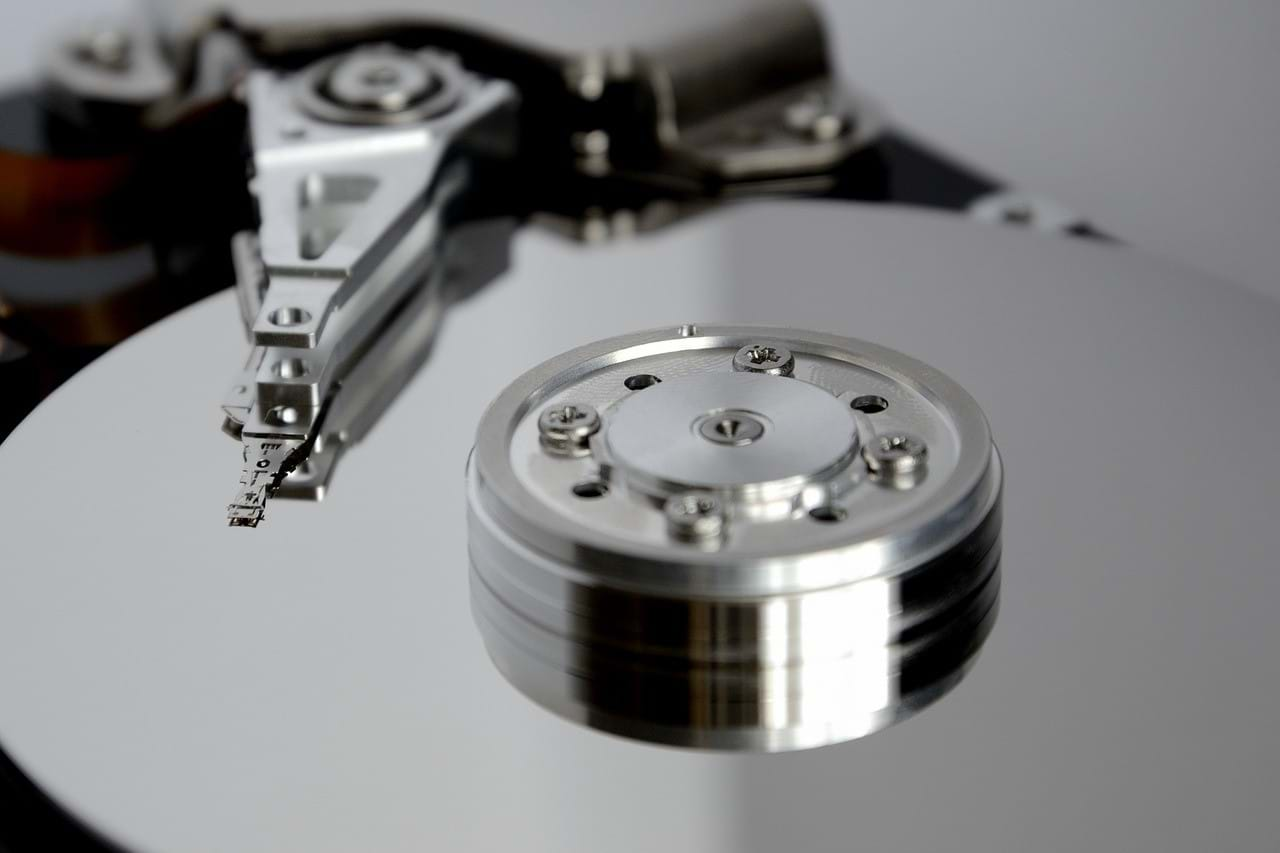﻿

# ExecuteScalar拿不到Update後的影響筆數？ExecuteNonQuery與ExecuteScalar的使用探討## 先建立基本心法，以免被下面的實驗搞混亂了

ExecuteNonQuery();

ExecuteScalar();

## 實驗環境程式碼

1. 資料庫名稱：TEST
2. 欄位一：iAutoIndex int 自動增量索引
3. 欄位二：cName NVarChar(50)

``````using (System.Data.SqlClient.SqlConnection oConn = new System.Data.SqlClient.SqlConnection("YourDB"))
{
using (System.Data.SqlClient.SqlCommand oCmd = new System.Data.SqlClient.SqlCommand())
{
oCmd.Connection = oConn;
oConn.Open();
oCmd.CommandText = "";

object oTemp = oCmd.ExecuteScalar();
int iTemp = System.Convert.ToInt32(oTemp);

//可以縮寫成下方程式碼，比較精簡
//int iTemp = System.Convert.ToInt32(oCmd.ExecuteNonQuery());
//int iTemp = System.Convert.ToInt32(oCmd.ExecuteScalar());
}
}
``````

## 實驗：SQL Insert

``````INSERT INTO TEST (cName) VALUES (@cName)
``````
``````//很OK，回傳插入筆數
int iTemp = System.Convert.ToInt32(oCmd.ExecuteNonQuery());
``````
``````//不OK。回傳型別恆等於null
int iTemp = System.Convert.ToInt32(oCmd.ExecuteScalar());
``````

### 實驗：SQL Insert（插入後馬上取回Index、IDENTITY）

``````INSERT INTO TEST (cName) VALUES (@cName); SELECT SCOPE_IDENTITY();
``````
``````//不OK。依然取到插入筆數
int iTemp = System.Convert.ToInt32(oCmd.ExecuteNonQuery());
``````
``````//很OK。取得到最後的索引值編號
int iTemp = System.Convert.ToInt32(oCmd.ExecuteScalar());
``````

## 實驗：SQL Delete

``````DELETE FROM TEST WHERE iAutoIndex in (3, 4, 5)
``````
``````//很OK，回傳刪除筆數
int iTemp = System.Convert.ToInt32(oCmd.ExecuteNonQuery());
``````
``````//不OK。回傳型別恆等於null
int iTemp = System.Convert.ToInt32(oCmd.ExecuteScalar());
``````

## 實驗：SQL Update

``````Update TEST SET cName=@cName WHERE iAutoIndex in (9, 10)
``````
``````//很OK，回傳更新筆數
int iTemp = System.Convert.ToInt32(oCmd.ExecuteNonQuery());
``````
``````//不OK。回傳型別恆等於null
int iTemp = System.Convert.ToInt32(oCmd.ExecuteScalar());
``````

## 實驗：SQL Select

``````Select * From TEST WHERE iAutoIndex in (9, 10)
``````
``````//不OK。回傳值永遠恆等於「-1」
int iTemp = System.Convert.ToInt32(oCmd.ExecuteNonQuery());
``````
``````//很OK，回傳第一列（First Row）第一欄（First Column）的資料
int iTemp = System.Convert.ToInt32(oCmd.ExecuteScalar());
``````

## 實驗：SQL Select（使用聚合函數count();）

``````Select count(*) From TEST WHERE iAutoIndex in (9, 10)
``````
``````//不OK。回傳值永遠恆等於「-1」
int iTemp = System.Convert.ToInt32(oCmd.ExecuteNonQuery());
``````
``````//很OK，回傳count找到的加總筆數
int iTemp = System.Convert.ToInt32(oCmd.ExecuteScalar());
``````

## 實驗：SQL OUTPUT for Insert

``````INSERT INTO TEST (cName) OUTPUT INSERTED.cName VALUES (@cName)
``````
``````//很OK，回傳插入的cName字串值
string cTemp = System.Convert.ToString(oCmd.ExecuteScalar());
``````

## 實驗：SQL OUTPUT for Update

``````UPDATE TEST SET cName=@cName OUTPUT INSERTED.iAutoIndex WHERE iAutoIndex in (13, 14)
``````
``````//很OK，回傳插入的第一列（First Row）的iAutoIndex欄的資料，也就是「13」
int iTemp = System.Convert.ToInt32(oCmd.ExecuteScalar());
``````

## 實驗：SQL OUTPUT for Delete

``````DELETE FROM TEST OUTPUT DELETED.iAutoIndex WHERE iAutoIndex in (13, 14)
``````
``````//很OK，回傳刪除的第一列（First Row）的iAutoIndex欄的資料，也就是「13」
int iTemp = System.Convert.ToInt32(oCmd.ExecuteScalar());
``````

## 如何把SQL OUTPUT子句的資料列舉取出

``````var oReader = oCmd.ExecuteReader();